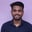Related Tags

python

# How to convert infix expressions to postfix expressions in PythonParmeshwar Patidar
Ambiguity in Infix notation

## Overview

Suppose * and + have the same operator precedence level:

• How would we interpret the infix expression A + B * C?

• What are the operands of *? Are they B and C or A+B and C?

This expression is ambiguous.

What if the infix expression is A + (B * C) or (A + B) * C? We can interpret it easily now because we know that the parenthesized expression is evaluated first.

We can remove ambiguity in the expression without using parentheses by converting infix expressions to postfix expressions.

### Postfix expression

In a postfix expression, operators follow their operands.

The postfix expression of A + B * C is A B C * +.

For example, in the A (B C *) + expression, * follows its operands, B C. Say B * C evaluates to K. Now, the expression becomes A K +. The + works the same way and follows its operands, A K.

Note: This shot implements the Shunting-yard algorithm. To Learn what the Shunting-yard algorithm is and how it works, see this shot: How to convert infix expressions to postfix.

### Code example

Here is the program that uses the Shunting-yard algorithm to convert an infix expression to postfix:

import re
# precedence level of supported operators.
PRECEDENCE = {
'^': 4, # highest precedence level
'*': 3,
'/': 3,
'+': 2,
'-': 2,
'(': 1,
}

def infixToPostfix(expr):
tokens = re.findall(r"(\b\w*[\.]?\w+\b|[\^\+\*\-\/])", expr)
stack = []
postfix = []

for token in tokens:
# If the token is an operand, then do not push it to stack.
# Instead, pass it to the output.
if token.isalnum():
postfix.append(token)

# If your current token is a right parenthesis
# push it on to the stack
elif token == '(':
stack.append(token)

# If your current token is a right parenthesis,
# pop the stack until after the first left parenthesis.
# Output all the symbols except the parentheses.
elif token == ')':
top = stack.pop()
while top != '(':
postfix.append(top)
top = stack.pop()

# Before you can push the operator onto the stack,
# you have to pop the stack until you find an operator
# with a lower priority than the current operator.
# The popped stack elements are written to output.
else:
while stack and (PRECEDENCE[stack[-1]] >= PRECEDENCE[token]):
postfix.append(stack.pop())
stack.append(token)

# After the entire expression is scanned,
# pop the rest of the stack
# and write the operators in the stack to the output.
while stack:
postfix.append(stack.pop())
return ' '.join(postfix)

# Let's convert infix to postfix

expressions = ['4*2+5*(2+1)/2', '4^2+5*(2+1)/2',  'A*(B+C)/D']

for expr in expressions:
print(infixToPostfix(expr))


The Program to convert an infix expression to a postfix expression

RELATED TAGS

python

CONTRIBUTORParmeshwar Patidar
RELATED COURSES

View all Courses

Keep Exploring

Learn in-demand tech skills in half the time